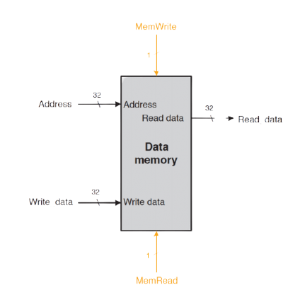## Memory in Verilog | Ram in Verilog# Memory in Verilog | Ram in VerilogMemory is a collection of storage cells referenced under common name. These cells are given continuous memory location in the system. Here each cell is the basic storage unit of memory. A basic storage unit of memory is termed as to a unit which can store one bit data.

Declaration of memory in verilog:

Firstly we should know how to declare a. Following is a code to show declaration of one bit memory cell.

reg r;

Here it is to be noted that we always term any kind of memory in Verilog as register, and reg is the keyword of register. The above code line will declare a memory cell of one bit named as ‘r’. Here we can store any single bit data in ‘r’. There would be some physical address of the cell but to access this cell in the program we will use name ‘r’, it is shown here,

r=0; Or r=1;

One Dimensional Memory:

Now we will discuss the case of one-dimensional memory where we have more than one cell. Here is a code showing the declaration of one-dimensional memory in a Verilog code.

reg [7:0]mem;

We referred it as one-dimensional as if we want to draw the memory we need to repeat the basic cell in one dimension only. The above code line will declare a block of memory cells with eight cells in the block. All these cells will be referenced under one common name ‘mem’. Here we have two ways to access ‘mem’ either by individually accessing each bit one at a time or by storing data of eight bits at once.

mem=1; mem=0; mem=0; mem=1; mem=0; mem=1; mem=1; mem=1;

Or mem=8’b11101001;

The former style is simple and need not to be explained. In later style the assignment is done by using three parameters. First is the number of bits to be stored here it is eight. Second is the alphabet which will tell the data is written in which format ‘b’ is for binary, ‘h’ is for hexadecimal and ‘d’ is for decimal. Now the third place is for data which is to be given in specified format.

Latest Posts

##### Effective Debugging Techniques in VHDL: Mastering the Art of Troubleshooting

Hot items

• 0.00

###### Resume Templates Mega Bundle For Electronics Engineers